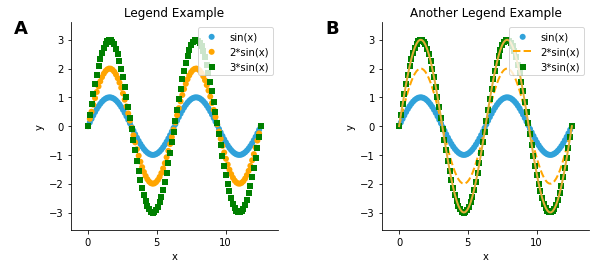# Legend Example#

URL: http://bokeh.pydata.org/en/latest/docs/gallery/legend.html

Most examples work across multiple plotting backends, this example is also available for:

```import numpy as np
import holoviews as hv

hv.extension('matplotlib')
```## Defining data#

```x = np.linspace(0, 4*np.pi, 100)
y = np.sin(x)

scatter1 = hv.Scatter((x, y), label='sin(x)')
scatter2 = hv.Scatter((x, y*2), label='2*sin(x)')
scatter3 = hv.Scatter((x, y*3), label='3*sin(x)')

curve1 = hv.Curve(scatter1)
curve2 = hv.Curve(scatter2)
curve3 = hv.Curve(scatter3)
```

## Plot#

```example1 = scatter1 * scatter2.opts(color='orange') * scatter3.opts(color='green')
example2 = (
scatter1 * curve1 * curve2.opts(color='orange', linestyle='--') *
scatter3.opts(color='green', marker='s') * curve3)

example1.relabel("Legend Example") + example2.relabel("Another Legend Example")
```This web page was generated from a Jupyter notebook and not all interactivity will work on this website. Right click to download and run locally for full Python-backed interactivity.